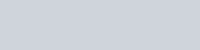# Lecture in Indefinite Integrals

(Last Updated On: December 13, 2017)“To integrate” is to find a function whose derivative is given. This process is called integration or anti-differentiation.
The term integral may also refer to the notion of the antiderivative, a function F whose derivative is the given function f.  In this case, it is called an indefinite integral and is written:$F = int f(x),dx.$

### Antiderivatives

Indefinite Integrals. If F is an antiderivative of a function f, i.e., F'(x) = f(x), then for any constant C, F(x) + C is another antiderivative of f(x).  The family of all antiderivatives of f is called indefinite integral of f and represented:
∫ f(x) dx = F(x) + C

Example: =

### Basic Integration Formulas(where n ≠ −1)### Integrating a Sum

Each term is integrated separately.### Constant Factor in the Integrand

A constant factor in the integrand can be written before the integral sign.where c is a constant

Again, Indefinite Integral is the family of functions that have a given function as a common derivative. The indefinite integral of f(x) is written: ∫ f(x) dx.

credit: Renato E. Apa-ap, et al.©2013 www.PinoyBIX.com